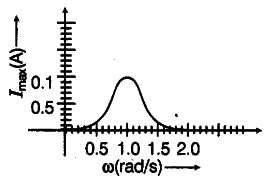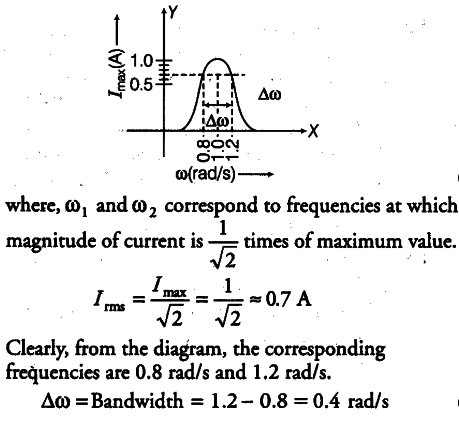# Find the bandwidth and mark in the figure

In series L- C- R circuit, the plot of { I }_{ max } versus ω is shown in the figure. Find the bandwidth and mark in the figure.Consider the diagram,
Bandwidth = { ω }_{ 2 } -
{ ω }_{ 1 }